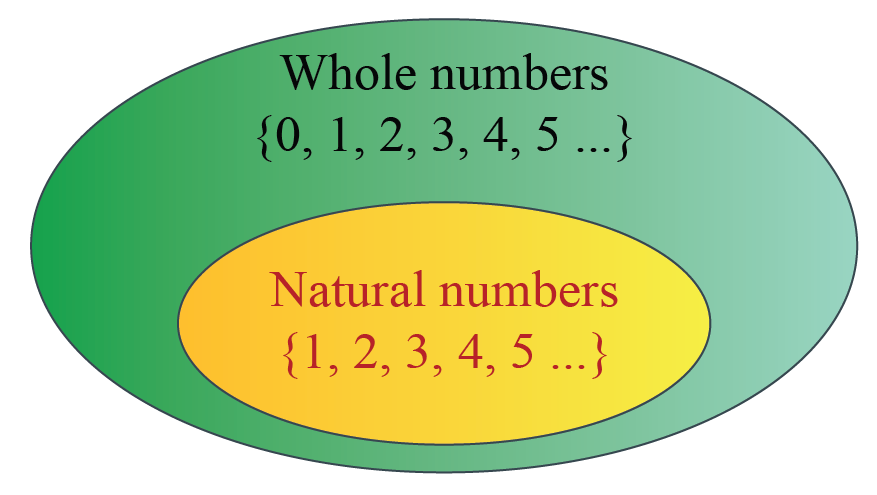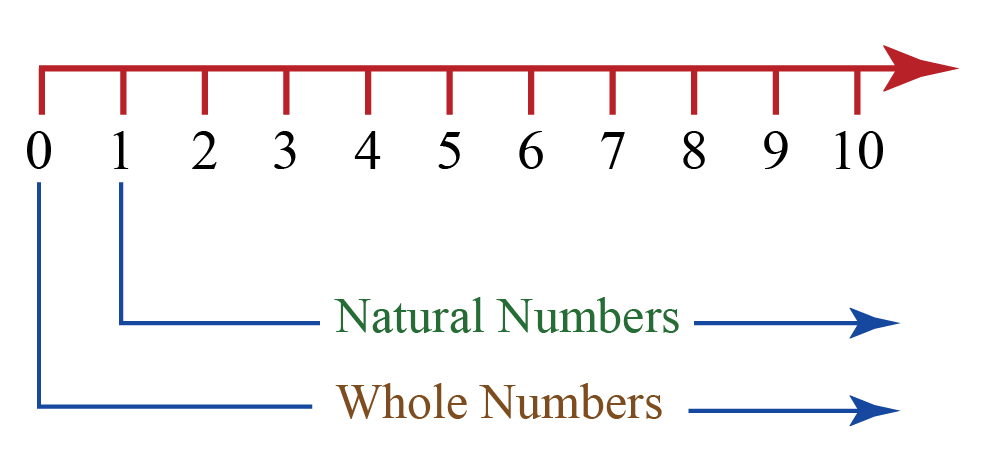# Whole Numbers

 1 Definition of a Natural Number 2 Definition of a Whole Number 3 Challenging Questions on Whole Numbers 4 Whole Numbers Vs Natural Numbers 5 Properties of Whole Numbers 6 Thinking out of the Box! 7 Solved Examples on Whole Numbers 8 Important Notes on Whole Numbers 9 Practice Questions on Whole Numbers 10 Important Topics of Whole Numbers 11 Frequently Asked Questions (FAQs)

We at Cuemath believe that Math is a life skill. Our Math Experts focus on the “Why” behind the “What.” Students can explore from a huge range of interactive worksheets, visuals, simulations, practice tests, and more to understand a concept in depth.

Book a FREE trial class today! and experience Cuemath’s LIVE Online Class with your child.

## Definition of a Natural Number

Natural numbers are the numbers that are used for counting.

The set of natural numbers in Mathematics is the set $$\textbf {1,2,3,...}$$

This set is denoted by the symbol $$N$$.

 N = $$\{1,2,3,4,5,...\}$$

Thus, the smallest natural number is $$1$$.

## Definition of a Whole Number

Whole Numbers are the set of natural numbers along with the number $$0$$.

The set of whole numbers in Mathematics is the set $$\textbf {0, 1,2,3,...}$$

This set of whole numbers is denoted by the symbol $$W$$.

 W = $$\{0,1,2,3,4…\}$$

In this set, the linear equation $$x + 2 = 2$$  is solvable because the solution of this equation is $$0$$,  which is a whole number.

Thus, going from the set $$N$$ to the set $${\text{W}}$$ has increased the solvability of equations.

Every equation which is solvable in $$N$$ is solvable in $${\text{W}}$$,

However, some equations which were not solvable in $$N$$ become solvable in $${\text{W}}$$.

### Whole Numbers Start from?

Whole numbers start from $$0$$ (from the definition of whole numbers).Challenging Questions
1. Which of the following statement is true?

a) Every natural number is a whole number.
b) Every whole number is a natural number.

## Whole Numbers Vs Natural Numbers

From the above definitions, we can understand that every whole number other than $$0$$ is a natural number.

Also, every natural number is a whole number.

So, the set of natural numbers is a part of the set of whole numbers.Both sets of numbers can be represented on the number line as follows:### Comparison between Whole numbers and Natural numbers

Whole Number Natural Number
The set of whole numbers is, $$W=\{0,1,2,3,...\}$$ The set of natural numbers is, $$N= \{1,2,3,...\}$$
The smallest whole number is $$0$$ The smallest natural number is $$1$$
We cannot determine the largest whole number We cannot determine the largest natural number
Each whole number is obtained by adding $$1$$ to its previous number Each natural number is obtained by adding $$1$$ to its previous number
$$0$$ is a whole number $$0$$ is NOT a natural number

## Properties of Whole Numbers

### 1. Closure Property:

The sum and product of two whole numbers is always a whole number.

The closure property of $$W$$ is stated as follows:

For all $$a,b \in W$$

 $a + b \in W\\ {\text{ and }} \\a \times b \in W$

### 2. Associative Property:

The sum or product of any three whole numbers remains the same though the grouping of numbers is changed.

The associative property of $$W$$ is stated as follows:

For all $$a,b,c \in W$$

 $\begin{gathered} a + \left( {b + c} \right) = \left( {a + b} \right) + c \\ {\text{and}} \\ a \times \left( {b \times c} \right) = \left( {a \times b} \right) \times c \\ \end{gathered}$

### 3. Commutative Property:

The sum and the product of two whole numbers remain the same even after interchanging the order of the numbers.

The commutative property of $$W$$ is stated as follows:

For all $$a,b \in W$$

 $\begin{gathered} a + b = b + a \\ {\text{and}} \\ a \times b = b \times a \\ \end{gathered}$

Let us summarise these three properties of whole numbers in a table.

Operation Closure Property Associative Property Commutative Property
Subtraction no no no
Multiplication yes yes yes
Division no no no

### 4. Distributive Property:

The distributive property of multiplication over addition is

 \begin{align}a \times (b+c) &= a \times b + a \times c\end{align}

The distributive property of multiplication over subtraction is

 \begin{align}a \times (b-c) = a \times b - a \times c\end{align}Think Tank
1. Consider the equation $$x + 2 = 1$$

We see that this equation is not solvable in $${\text{W}}$$. In which number system will it be solvable?

2. Is $$W$$ closed under subtraction and division?

3. Is $$W$$ associative under subtraction and division?

4. Is $$W$$ commutative under subtraction and division?

Help your child score higher with Cuemath’s proprietary FREE Diagnostic Test. Get access to detailed reports, customised learning plans and a FREE counselling session. Attempt the test now.

## Solved Examples

 Example 1

Identify the whole numbers among the following numbers.

$$-1, 0, 3, \dfrac{1}{2}, 5$$.

Solution:

The set of whole numbers in Mathematics is the set $$\textbf {0, 1, 2, 3, ...}$$

So, among the given numbers, the whole numbers are, $$0, 3 \text{ and }5$$.

 Example 2

Identify the whole numbers among the following numbers.

$$7, 2, -3, -\dfrac{3}{5}, 0$$.

Solution:

The set of whole numbers in Mathematics is the set $$\textbf {0, 1, 2, 3, ...}$$

So, among the given numbers, the whole numbers are, $$7,2, \text{ and }0$$.

Numbers and Number Systems
Numbers and Number Systems
grade 9 | Questions Set 2
Numbers and Number Systems
grade 9 | Questions Set 1
Numbers and Number Systems